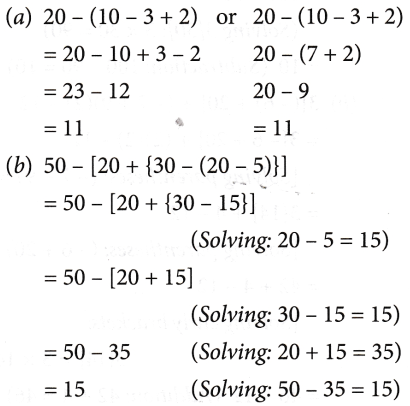## Decimal Representation Of Rational Numbers

Example 1:    Express $$\frac { 7 }{ 8 }$$ in the decimal form by long division method.
Solution:    We have,∴ $$\frac { 7 }{ 8 }$$ = 0.875

Example 2:    Convert $$\frac { 35 }{ 16 }$$ into decimal form by long division  method.
Solution:    We have,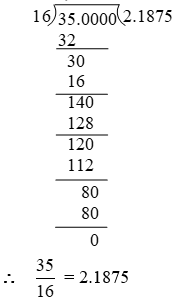Example 3:    Express $$\frac { 2157 }{ 625 }$$ in the decimal form.
Solution:    We have,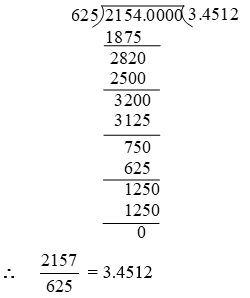Example 4:    Express $$\frac { -17 }{ 8 }$$ in decimal form by long division method.
Solution:    In order to convert $$\frac { -17 }{ 8 }$$ in the decimal form, we first express $$\frac { 17 }{ 8 }$$ in the decimal form and the decimal form of $$\frac { -17 }{ 8 }$$ will be negative of the decimal form of $$\frac { 17 }{ 8 }$$
we have,Example 5:    Find the decimal representation of $$\frac { 8 }{ 3 }$$ .
Solution:    By long division, we haveExample 6:    Express $$\frac { 2 }{ 11 }$$ as a decimal fraction.
Solution:    By long division, we haveExample 7:    Find the decimal representation of $$\frac { -16 }{ 45 }$$
Solution:    By long division, we haveExample 8:    Find the decimal representation of $$\frac { 22 }{ 7 }$$
Solution:    By long division, we have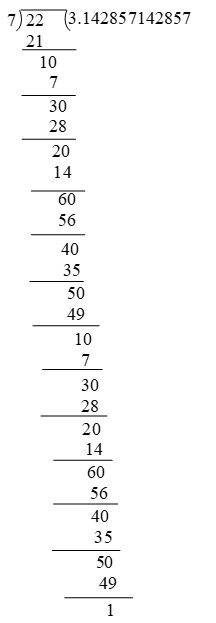So division of rational number gives decimal expansion. This expansion represents two types
(A) Terminating (remainder = 0)So these are terminating and non repeating (recurring)
(B) Non terminating recurring (repeating)
(remainder ≠ 0, but equal to devidend)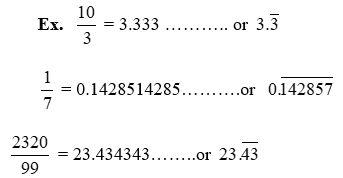These expansion are not finished but digits are continusely repeated so we use a line on those digits, called bar $$(\bar{a})$$.
So we can say that rational numbers are of the form either terminating, non repeating or non terminating repeating (recurring).

## What is a Ratio and Proportion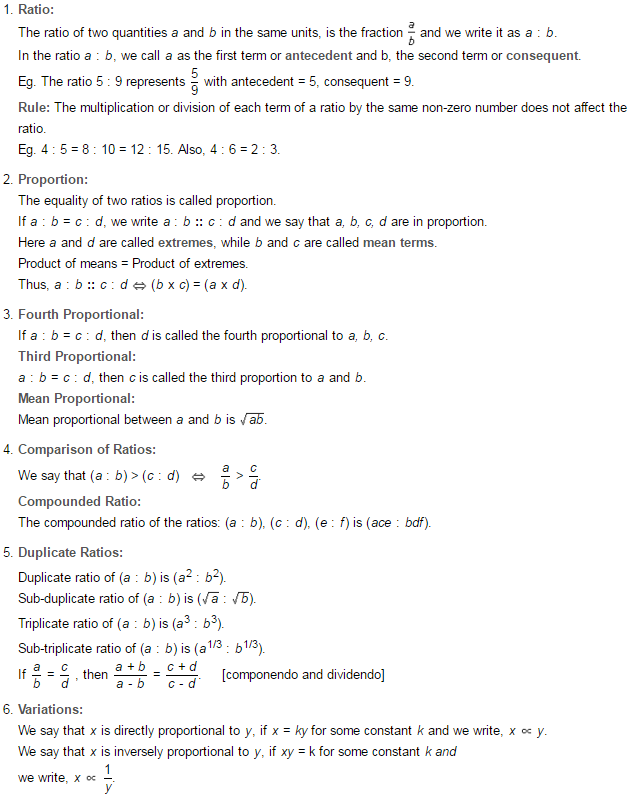RATIO
In our day-to-day life, we compare one quantity with another quantity of the same kind by using the ‘method of subtraction’ and ‘method of division’.
Example: The height of Seema is 1 m 67 cm and that of Reema is 1 m 62 cm. The difference in their heights is:
167 cm – 162 cm = 5 cm
Thus, we say Seema is 5 cm taller than Reema.
Similarly, suppose the weight of Seema is 60 kg and the weight of Reema is 50 kg. We can compare their weights by division, i.e.,
$$\frac{\text{Weight of Seema}}{\text{Weight of Reema}}=\frac{\text{50 kg}}{\text{60 kg}}$$
$$=\frac { 6 }{ 5 }$$
So, the weight of Seema is $$\frac { 6 }{ 5 }$$ times the weight of Reema.
When we compare two similar quantities by division, the comparison is called the ‘ratio’. It is denoted by ‘:’ and read as ‘is to’.
Example: $$\frac { 5 }{ 8 }$$ = 5 : 8 (read as 5 is to 8).
As shown in the above example a ratio is like a fraction or comparison of two numbers, where a numerator and a denominator is separated by a colon (:). The first term or the quantity (5), called antecedent means ‘that precedes’ and the second term, called consequent means ‘that follows’.

### Properties of Ratio

When we compare two quantities, the following points must be taken care of:

1. A ratio is usually expressed in its simplest form.
Example:
$$\frac{12}{36}=\frac{1}{3}=1:3$$
2. Both the quantities should be in the same unit. So, ratio is a number with no unit involved in it.
Example: 200 g : 2 kg
= 200 g : 2000 g
$$\frac{200}{2000}=\frac{1}{10}=1:10$$
3. The order of the quantities of a ratio is very important.
Example: 5 : 6 is different from 6 : 5.
They are not equal.
5 : 6 ≠ 6 : 5

### Equivalent Ratios

A ratio is similar to a fraction. So, if we divide or multiply the numerator (antecedent) and denominator (consequent) by the same number, we get an equivalent fraction (ratio).
Example: 5 : 6 = $$\frac { 5 }{ 6 }$$

### Comparison of Ratios

To compare two ratios, we have to follow these steps:
Step 1: Convert each ratio into a fraction in its simplest form.
Step 2: Find the LCM of denominators of the fractions obtained in step 1.
Step 3: Convert the denominators equal to LCM obtained in step 2 in each fraction.
Step 4: Now, compare the numerators of the fractions; the fraction with a greater numerator will be greater than the other.

Example 1: Compare the ratio 5: 6 and 7: 9.
Solution:
Express the given ratios as fraction
5 : 6 = $$\frac { 5 }{ 6 }$$ and 7 : 9 = $$\frac { 7 }{ 9 }$$
Now find the L.C.M (least common multiple) of 6 and 9
The L.C.M (least common multiple) of 6 and 9 is 18.
Making the denominator of each fraction equal to 18, we have
$$\frac { 5 }{ 6 }$$ = (5 ×3)/(6 ×3) = $$\frac { 15 }{ 18 }$$ and $$\frac { 7 }{ 9 }$$ = (7 ×2)/(9 ×2) = $$\frac { 14 }{ 18 }$$
Clearly, 15 > 14
Now, $$\frac { 15 }{ 18 }$$ > $$\frac { 14 }{ 18 }$$
Therefore, 5 : 6 > 7 : 9.

Example 2: Convert the ratio 275 : 125 in its simplest form.
Solution:
Find the GCD (or HCF) of numerator and denominator
GCD of 275 and 125 is 25
Divide both the numerator and denominator by the GCD
$$\frac{275 \div 25}{125 \div 25}$$
Reduced fraction:
$$\frac { 11 }{ 5 }$$
Therefore, $$\frac { 275 }{ 125 }$$ simplified to lowest terms is $$\frac { 11 }{ 5 }$$.

Example 3: Write the following ratios in descending order:
1 : 3, 5 : 12, 4 : 15 and 2 : 3
Solution: We have,Example 4: Mr Lai divides a sum of Rs. 1500 between his two sons in the ratio 2 : 3. How much money does each son get?
Solution:
Let the first son get 2x and the second son get 3x.
⇒ 2x + 3x = 1500
⇒ 5x = 1500
⇒ x = $$\frac { 1500 }{ 5 }$$
⇒ x = 300
∴ first son get 2x = 2 × 300 = 600
second son get 3x = 3 × 300 = 900

Example 5: Two numbers are in the ratio 3 : 5 and their sum is 96. Find the numbers.
Solution: Let the first number be 3x and the second number be 5x.
Then, their sum = 3x + 5x = 96
8x = 96
x = 12
The first number = 3x = 3 × 12 = 36
The second number 5x = 5 × 12 = 60

Example 6: In a pencil box there are 100 pencils. Out of which 60 are red pencils and the rest are blue pencils. Find the ratio of:
(a) blue pencils to the total number of pencils.
(b) red pencils to the total number of pencils.
(c) red pencils to blue pencils.
Solution: Total number of pencils in the pencil box = 100
(a) no. of blue pencil = 100 – 60 = 40
no. of total pencil = 100
Ratio = $$\frac { 40 }{ 100 }$$ =$$\frac { 4 }{ 10 }$$ =$$\frac { 2 }{ 5 }$$
(b) no. of red pencil = 60
no. of blue pencil = 40
Ratio = $$\frac { 60 }{ 40 }$$ =$$\frac { 6 }{ 4 }$$ =$$\frac { 3 }{ 2 }$$
(c) no. of red pencil = 60
no. of total pencil = 100
Ratio = $$\frac { 60 }{ 100 }$$ =$$\frac { 6 }{ 10 }$$ =$$\frac { 3 }{ 5 }$$

PROPORTION
A proportion is an equation with a ratio on each side. It is a statement that two ratios are equal. When two ratios are equal then such type of equality of ratios is called proportion and their terms are said to be in proportion.

Example: If the cost of 3 pens is Rs. 21, and that of 6 pens is Rs. 42, then the ratio of pens is 3 : 6, and the ratio of their costs is 21 : 42. Thus, 3 : 6 = 21 : 42. Therefore, the terms 3, 6, 21, and 42 are in proportion.
Generally, the four terms, a, b, c, and d are in proportion if a : b = c : d.
Thus, a : b : : c : d means  a/b = c/d or ad = ad = bc
Conversely, if ad = be, then a/b = c/d  or a : b : : c : d
Here, a is the first term, b is the second term, c is the third term, and d is the fourth term. The first and the fourth terms are called extreme terms or extremes and the second and third terms are called middle terms or means.

Continued proportion
In a proportion, if the second and third terms are equal then the proportion is called continued proportion.
Example: If 2 : 4 : : 4 : 8, then we say that 2, 4, 8 are in continued proportion.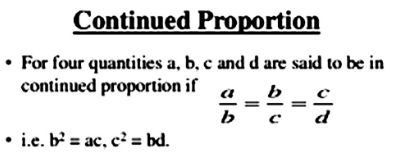Mean proportion
If the terms a, b, and c are in continued proportion, then ‘b’ is called the mean proportion of a and c.
Example: If a, b, c are in continued proportion, then
Mean proportion = b2 = ac

Third proportion
If the terms a, b, c are in continued proportion, then c is called the third proportion.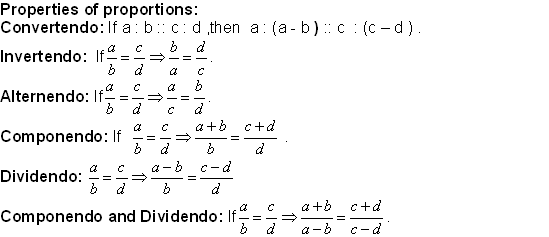Example 1: Find x, where x : 3 : : 4 : 12.
Solution: Here, x, 3, 4, and 12 are in proportion.
$$\frac { x }{ 12 }$$ = $$\frac { 3 }{ 4 }$$
Now, after cross multiplying (the denominator of L.H.S. gets multiplied to the numerator of R.H.S. and similarly the denominator of R.H.S. with the numerator of L.H.S.), we get
4 × x = 3 × 12
4 × x = 36
x = $$\frac { 36 }{ 4 }$$
x = 9
So, the answer is x = 9.

Example 2: Find the third proportion of 10 and 20.
Solution: If a, b, c are in proportion, then b2 = ac.
10:20 = 20:x
$$\frac { 10 }{ 20 }$$ = $$\frac { 20 }{ x }$$
20 × 20 = 10 × x
x = 40

Example 3: Find the value of x, if 14, 42, x are in continued proportion.
Solution: Here 14, 42, and x are in proportion.
The numbers is the continued proportion are in the form of $$\frac { a }{ b }$$ = $$\frac { b }{ c }$$
Here, a = 14, b = 42 and c = x
Cross multiply
14 × x = 42 × 42
14 × x = 1764
∴ x = 126
∴ The value of x is 126.

Example 4: The cost of 1 dozen bananas is Rs. 24. How much do 50 bananas cost?
Solution: Let the cost of 50 bananas be x.
cost of 12 bananas =₹24
cost of 1 banana = $$\frac { 24 }{ 12 }$$ = ₹2
cost of 50 bananas = (50 ×2) = ₹100

Example 5: Rajesh drives his car at a constant speed of 12 km per 10 minutes. How long will he take to cover 48 km?
Solution: Let Rajesh take x mins, to cover 48 km.
12 : 10
48 : x
⇒ 12 × x = 48 × 10
x = 40
therefore it will take Rajesh 40min to travel 48km.

## What is the pattern of numbers?

PATTERNS
Patterns arise due to special arrangements of numbers associated with geometrical figures. The numbers are of different kinds like

1. Natural numbers
2. Polygonal numbers
(a) Triangular numbers
(b) Square numbers
(c) Pentagonal numbers
(d) Hexagonal numbers, etc.

Examples

1. Patterns in natural numbers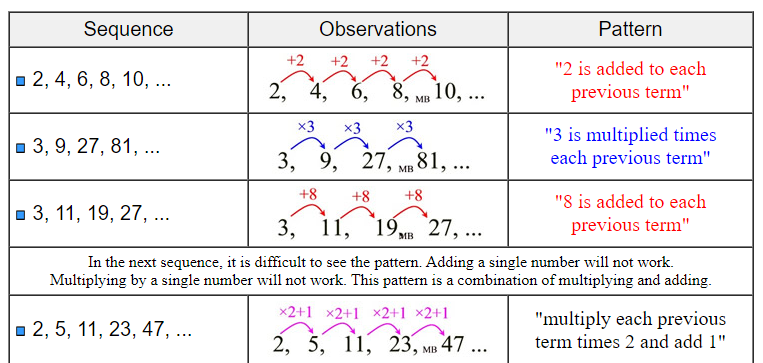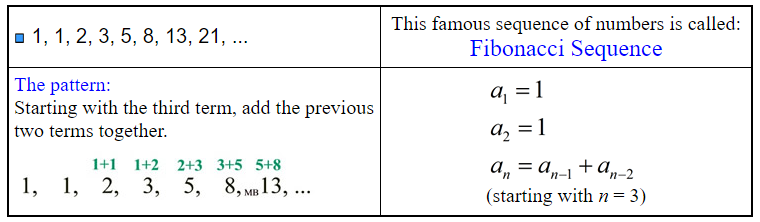2. Patterns in polygonal numbers

(a) Triangular numbers: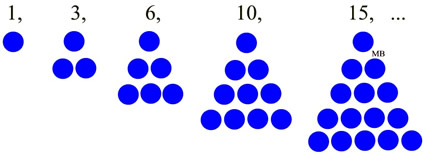Here the numbers of dots up to any stage are given by the pattern
1, 3, 6, 10, 15, ……
These numbers arise from triangles and so these are called triangular numbers.

(b) Square numbers: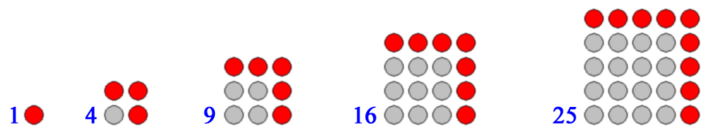Here the numbers of dots upto any stage are given by the pattern
1, 4, 9, 16, 25, 36, ……
These numbers arise from squares and are called square numbers.

## Whole Numbers And Its Properties

WHOLE NUMBERS
Now if we add zero (0) in the set of natural numbers, we get a new set of numbers called the whole numbers. Hence the set of whole numbers consists of zero and the set of natural numbers. It is denoted by W. i.e., W = {0, 1, 2, 3, . . .}. Smallest whole number is zero.

### Properties of whole numbers

All the properties of numbers satisfied by natural numbers are also satisfied by whole numbers. Now we shall learn some fundamental properties of numbers satisfied by whole numbers.

### Properties of Addition

(a) Closure Property: The sum of two whole numbers is always a whole number. Let a and b be two whole numbers, then a + b = c is also a whole number.
This property is called the closure property of addition

Example: 1 + 5 = 6 is a whole number.

(b) Commutative Property: The sum of two whole numbers remains the same if the order of numbers is changed. Let a and b be two whole numbers, then
a + b = b + a
This property is called the commutative property of addition.

(c) Associative Property: The sum of three whole numbers remains the same even if the grouping is changed. Let a, b, and c be three whole numbers, then
(a + b) + c = a + (b + c)
This property is called the associative property of addition.

(d) Identity Element: If zero is added to any whole number, the sum remains the number itself. As we can see that 0+a=a=a+0 where a is a whole number.
Therefore, the number zero is called the additive identity, as it does not change the value of the number when addition is performed on the number.

### Properties of Subtraction

(a) Closure Property: The difference of two whole numbers will not always be a whole number. Let a and b be two whole numbers, then a – b will be a whole number if a > b or a = b. If a < b, then the result will not be a whole number. Hence, closure property does not hold good for subtraction of whole numbers.
Examples
17 – 5 = 12 is a whole number.
5 – 17 = – 12 is not a whole number.

(b) Commutative Property: If a and b are two whole numbers, then a – b ≠ b – a. It shows that subtraction of two whole numbers is not commutative. Hence, commutative property does not hold good for subtraction of whole numbers, i.e.,
a – b ≠ b – a.
Example: 3 – 4 = – 1 and 4 – 3 = 1
∴ 3 – 4 ≠ 4 – 3

(c) Associative Property: If a, b, and c are whole numbers, then (a – b) – c ≠ a – (b – c). It shows that subtraction of whole numbers is not associative. Hence, associative property does not hold good for subtraction of whole numbers.
Example: (40 – 25) – 10 = 15 – 10 = 5
40 – (25 – 10) =40- 15 = 25
∴ (40 – 25) – 10 ≠ 40 – (25 – 10)

(d) Property of Zero: If we subtract zero from any whole number, the result remains the number itself.
Example: 7 – 0 = 7
5 – 0 = 5

### Properties of Multiplication

(a) Closure Property: If a and b are two whole numbers, then a × b = c will always be a whole number. Hence, closure property holds good for multiplication of whole numbers.
Example: 5 × 7 = 35 (a whole number)
6 × 1 = 6 (a whole number)

(b) Commutative Property: If a and b are two whole numbers, then the product of two whole numbers remains unchanged if the order of the numbers is interchanged, i.e.,
a × b = b × a.
Example: 6 × 5 = 5 × 6
30 = 30
i. e.,  6 rows of 5 or 5 rows of 6 give the same results.
so, 6 × 5 = 30 = 5 × 6

(c) Associative Property: If a, b, and c are whole numbers, then the product of three whole numbers remains unchanged even if they are multiplied in any order. Hence, associative property does hold good for multiplication of whole numbers, i.e.,
(a × b) × c = a × (b × c)
Example:
(4 × 5) × 8 = 4 × (5 × 8)
20 × 8 = 4 × 40
160 = 160

(d) Multiplicative Identity: If any whole number is multiplied by 1, the product remains the number itself. Let a whole number be a, then
a × 1 = a = 1 × a.
3 × 1 = 3 = 1 × 3
Examples
75 × 1 = 75 = 1 × 75
3 × 1 = 3 = 1 × 3
Hence, 1 is called the multiplicative identity.

(e) Multiplicative Property of Zero: Any whole number multiplied by zero gives the product as zero.If a is any whole number, then 0 × a = a × 0 = 0.
Example: 3 × 0 = 0 × 3 = 0

### Properties of Division

(a) Closure Property: If a and b are whole numbers, then a ÷ b is not always a whole number. Hence, closure property does not hold good for division of whole numbers.
Example: 7 ÷ 5 = $$\frac { 7 }{ 5 }$$ is not a whole number.
7 ÷ 7 = 1 is a whole number.

(b) Commutative Property: If a and b are whole numbers, then a ÷ b ≠ b ÷ a. Hence, commutative property does not hold good for division of whole numbers.
Example: 18 ÷ 3 = 6 is a whole number.
3 ÷ 18 = $$\frac { 3 }{ 18 }$$ = $$\frac { 1 }{ 6 }$$  is not a whole number.
∴ 3 ÷ 18 ≠ 18 ÷  3

(c) Associative Property: If a, b, and c are whole numbers then (a ÷ b) ÷  c ≠ a ÷  (b ÷  c). Hence, associative property does not hold good for division of whole numbers.

Example: (15 ÷  3) ÷ 5 = 5 ÷  5 = 1
15 ÷  (3 ÷  5) = 15 ÷  3/5 = 15 × 5/3
= 25
∴ (15 ÷  3) ÷ 5 ≠ 15 ÷  (3 ÷  5)

(d) Property of Zero: If a is a whole number then 0 ÷ a = 0 but a ÷ 0 is undefined.
Example: 6 ÷ 0 is undefined.

Note:

• Product of zero and a whole number gives zero.
a × 0 = 0
• Zero divided by any whole number gives zero.
0 ÷ a = 0
a ÷ 0 = undefined
• Any number divided by 1 is the number itself.
a ÷ 1 = a

DISTRIBUTIVE PROPERTY

You are distributing something as you separate or break it into parts.
Example: Raj distributes 4 boxes of sweets. Each box comprises 6 chocolates and 10 candies. How many sweets are there in these 4 boxes?
∴ Chocolates in 1 box = 6
Chocolates in 4 boxes = 4 × 6 = 24
Candies in 1 box =10
Candies in 4 boxes = 4 × 10 = 40
Total number of sweets in 4 boxes
= 4 × 6 + 4 × 10 = 4 × (6 + 10)
= 4 × 16 = 64

Hence, we conclude the following:
(a) Multiplication distributes over addition, i.e., a(b + c) = ab + ac, where a, b, c are whole numbers.
Example: 10 × (6 + 5) = 10 × 6 + 10 × 5
10 × 11 = 60 + 50
110 = 110
This property is called the distributive property of multiplication over addition.
(b) Similarly, multiplication distributes over subtraction, i.e., a × (b – c) = ab – ac where a, b, c are whole numbers and b > c.
Example: 10 × (6 – 5) = 10 × 6 – 10 × 5
10 × 1 = 60 – 50
10 = 10
This property is called the distributive property of multiplication over subtraction.

Example 1: Determine the following by suitable arrangement.
2 × 17 × 5
Solution: 2 × 17 × 5 = (2 × 5) × 17
= 10 × 17 = 170

Example 2: Solve the following using distributive property.
97 × 101
Solution: 97 × 101 = 97 × (100 + 1)
= 9700 + 97 = 9797

Example 3: Tina gets 78 marks in Mathematics in the half-yearly Examination and 92 marks in the final Examination. Reena gets 92 marks in the half- yearly Examination and 78 marks in the final Examination in Mathematics. Who has got the higher total marks?
Solution: Tina gets the following marks = 78 + 92 = 170 Total marks
Reena gets the following marks = 92 + 78 = 170 Total marks
So, both of them got equal marks.

Example 4: A fruit seller placed 12 bananas, 10 oranges, and 6 apples in a fruit basket. Tarun buys 3 fruit baskets for a function. What is the total number of fruits in these 3 baskets?
Solution: Number of bananas in 3 baskets = 12 × 3 = 36 bananas
Number of oranges in 3 baskets = 10 × 3 = 30 oranges
Number of apples in 3 baskets = 6×3 = 18 apples
Total number of fruits = 36 + 30+ 18 = 84
Alternative Method
Total number of fruits in 3 baskets
= 3 × [ 12 +(10 + 6)]
= 3 × [ 12 + 16]
= 3 × 28 = 84

### Representation Of Whole Numbers On A Number Line

We can represent whole numbers-on a straight line. To represent a set of whole numbers on a number line, let’s first draw a straight line and mark a point O on it. After that, mark points A, B, C, D, E, F on the line at equal distance, on the right side of point O.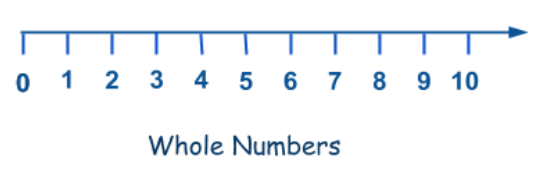Now, OA = AB = BC = CD and so on
Let OA = 1 unit
OB = OA + AB = 1 + 1 = 2 units
OC = OB + BC = 2 + 1 = 3 units
OD = OC + CD = 3 + 1 = 4 units and so on.
Let the point O correspond to the whole number 0, then points A, B, C, D, E, …..  correspond to the whole numbers 1, 2, 3, 4, 5,…. In this way every whole number can be represented on the number line.

## OPERATIONS ON NUMBERS

Addition of two or more numbers gives us another number. The numbers which are added are called addends and the new number thus obtained is called the sum. For example,
34670 + 12345 = 47015
Here, 34670 and 12345 are called addends and 47015 is called the sum of 34670 and 12345. Large numbers are added in the same way as the small numbers.

### Addition by grouping

When we add three numbers, we can add any two numbers first and then add the third number to the sum. In other words, we can group any two of the three numbers in order to find the sum of the three numbers. If we have to add more than three numbers, we can similarly group any two of the given numbers in several ways and add them. For example, suppose we want to add 234523, 123098, 555623, and 876543. We can find the sum in any of the following ways:

• [(234523 + 123098) + 555623] + 876543
= (357621 + 555623) + 876543
= 913244 + 876543 = 1789787
• [(234523 + 123098) + 876543] + 555623
= (357621 + 876543) + 555623
= 1234164 + 555623 = 1789787

So, we can add three or more numbers by grouping them in any way we feel convenient.

### Subtraction

Subtraction of one number from another number gives us a third number. The new number thus obtained is called the difference of the two numbers. For example,
70000 – 67429 =2571
Here, 2571 is the difference of 70000 and 67429. Large numbers are subtracted in the same way as the small numbers.

### Multiplication

Multiplication of two or more numbers gives us another number. The new number thus obtained is called the product of those numbers. For example, 11 × 13 = 143.
Here, 143 is the product of 11 and 13. 11 and 13 are called the/actors of 143. Note that 1 is always a factor of any number.
Let us take another example, say, 855 × 73 = 62415. Here, 855 and 73 are factors of 62415, and 62415 is the product of 855 and 73.
Large numbers are multiplied in the same way as the small numbers.

### Multiplication using zeros

When we multiply a number by 10,100,1000, etc. we simply place those many zeros on the right of that number. For example, if we want to multiply 15 by 10, then the answer will be 150. Similarly, if we want to multiply 15 by 100, then the answer will be 1500, and so on. Zeros have been added on the right side of 15.

### Division

Division of a number by another number gives two new numbers—the quotient and the remainder. The number that is divided is called the dividend and the number that divides is called the divisor. For example,Here, 7 is the divisor; 66 is the
dividend; 9 is the quotient, and 3 is the remainder.

Divide 69205 by 432 and find the quotient and remainder.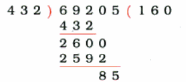Here, the quotient is 160 and remainder is 85.

### Mixed operations involving +, –, ×, and ÷

Till now we have solved problems involving only one type of operation, that is, one of the following: addition, subtraction, division, and multiplication. But what do we do if a problem involves two or more operations together? Consider the following problem:
Example: Simplify: 16 – 6 + 2 – 3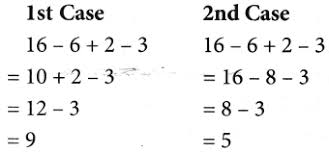In the 1st case, the answer is 9 and in the 2nd case, it is 5. We get different answers depending on the order in which the operations are carried out. But one of the two answers we got is wrong. In order to avoid this kind of ambiguity, an international convention has been accepted.

1. If any mathematical expression has symbols of addition and subtraction both, we first add and then subtract. For example, consider the following case: 16 – 3 + 4 – 5
= 20 – 3 – 5    (Addition: 16 + 4 = 20)
= 17 – 5            (Subtraction: 20 – 3 = 17)
= 12                  (Subtraction: 17 – 5 = 12)
2. If in three operations [(+, – , and × ) or (+, – , and ÷)], that is, besides addition and subtraction, a problem involves multiplication or division, we first multiply or divide, and then go for addition and subtraction respectively. For example, consider the following cases.
(a) Simplify: 7 + 3 × 4 – 3
In the above example, the three operations involved are +, – , and ×. To solve this problem, we first multiply the numbers, then we go for addition, and at the end we subtract.
7 + 3 × 4 – 3
= 7 + 12 – 3 (Multiplication: 3 × 4 = 12)
= 19 – 3        (Addition: 7 + 12 = 19)
= 16               (Subtraction: 19 – 3 = 16)
(b) Simplify: 16 – 6 ÷ 2 + 8
In the above example, the three operations involved are +, – , and ÷. To solve this problem, we first divide, then add, and at the end subtract the number. 16 – 6 ÷ 2 + 8
= 16 – 3 + 8  (Division: 6 ÷ 2 = 3)
= 24 – 3        (Addition: 16 + 8 = 24)
= 21               (Subtraction: 24 – 3 = 21)
3. When a problem involves all the operations namely, +, – , × , and ÷, then there is an agreed formula denoted by ‘DMAS’, which mathematicians follow. In ‘DMAS’, D stands for division, M for multiplication, A for addition, and S for subtraction. DMAS represents the order of operations. For example, consider the following cases.
(a) Simplify: 5 + 4 × 3 – 9 ÷ 3
In the above example, all the four operations are there, so we have to use DMAS rule, as shown below: 5 + 4 × 3 – 9 ÷ 3
= 5 + 4 × 3 – 3 (Division: 9 ÷ 3 = 3)
= 5 + 12 – 3       (Multiplication: 4 × 3 = 12)
= 17 – 3              (Addition: 5 + 12 = 17)
= 14                     (Subtraction: 17 – 3 = 14)
(b) Simplify: 7 × 3 – 4 + 60 ÷ 10
In this example too, all the four operations are there, hence to simplify this we have to use DMAS rule. 7 × 3 – 4 + 60 ÷ 10
= 7 × 3 – 4 + 6 (Division: 60 ÷ 10 = 6)
= 21 – 4 + 6      (Multiplication: 7 × 3 = 21)
= 27 – 4             (Addition: 21 + 6 = 27)
= 23                    (Subtraction: 27 – 4 = 3)

### Using the operation ‘Of’

Sometimes we need to find the value of ‘$$\frac { 1 }{ 2 }$$ of 16’ or ‘3 of 5’.
This means we need to find the value of $$\frac { 1 }{ 2 }$$ × 16 or 3 × 5.
So ‘of’ means multiplication.
Hence, $$\frac { 1 }{ 2 }$$ of 16 = $$\frac { 1 }{ 2 }$$ × 16
= 8
and 3 of 5 =3 × 5
= 15.
When the operation ‘of’ appears in any mathematical expression, then it must be performed before any other operation. To solve such kind of expression we use ODMAS rule, in which O stands for of, D for division, M for multiplication, A for addition, and S for subtraction.
Consider the following examples.

Example 1: Simplify 36 ÷ 2 of 3 + 6 × 2.
Solution: To solve this, we first solve the operation ‘of’.
36 ÷ 2 of 3 + 6 × 2
= 36 ÷ 6 + 6 × 2  (Of. 2 of 3 = 2 × 3 = 6)
= 6 + 6 × 2           (Division: 36 ÷ 6 = 6)
= 6 + 12                (Multiplication: 6 × 2 = 12)
= 18                       (Addition: 6 + 12 = 18)

Example 2: Simplify 42 ÷ 6 × 2 + $$\frac { 1 }{ 7 }$$ of 35 × 2.
Solution: 42 ÷ 6 × 2 + $$\frac { 1 }{ 7 }$$ of 35 × 2
= 42 ÷ 6 × 2 + 5 × 2  (Of: $$\frac { 1 }{ 7 }$$ of 35 = $$\frac { 1 }{ 7 }$$ × 35 = 5)
= 7 × 2 + 5 × 2           (Division: 42 ÷ 6 = 7)
= 14 + 10                    (Multiplication: 7 × 2 = 14 and 5 × 2 = 10)
= 24                            (Addition: 14 + 10 = 24)

### Use of brackets and the BODMAS rule

Let us consider an example to illustrate the use of brackets.
Rima bought 35 chocolates and ate 5 of them. She distributed the remaining chocolates equally among 6 of her friends. How many chocolates did she give to each of them?

In this problem we have to subtract 5 chocolates that Rima ate, from 35 chocolates she had, before dividing them among 6 of her friends. So we have to first perform the operation of subtraction and then do division. In such cases, we use brackets around the part that has to be done first, that is(35 – 5) ÷ 6 (First solve bracket, i.e., 35 – 5 = 30)
= 30 ÷ 6      (Division: 30 ÷ 6 = 5)
= 5
Consider another example.

Example 3: Solve 2 of 3 × (5 + 2).
Solution: 2 of 3 × (5 + 2)
= 2 of 3 × 7  (First bracket: 5 + 2 = 7)
= 6 × 7          (Of: 2 of 3 = 2 × 3 = 6)
= 42              (Multiplication: 6 × 7 = 42)
Hence, when problems involve brackets, of, ×, ÷, +, and – then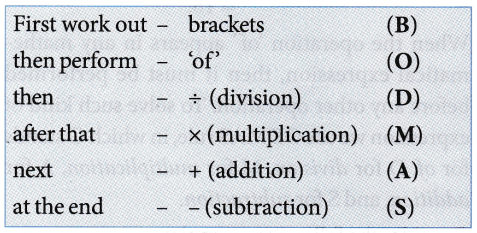To make it easy to remember this order, we remember the word BODMAS, where B stands for brackets, O for of’, D for division, M for multiplication, A for addition, and S for subtraction. This is called the ‘BODMAS‘ rule.
Sometimes numerical expressions may involve different types of brackets. These brackets are

• Vinculum or bar —
• Parentheses or small brackets ( )
• Braces or curly brackets { }
• Square brackets or big brackets [ ]

We simplify expressions by starting with the innermost bracket. Usually the vinculum is the innermost bracket, next is the parentheses, then the braces, and finally the square brackets. Let us now consider some examples.

Example 4: Simplify $$25-[20-\{10-(7-\overline{5-3})\}]$$.
Solution: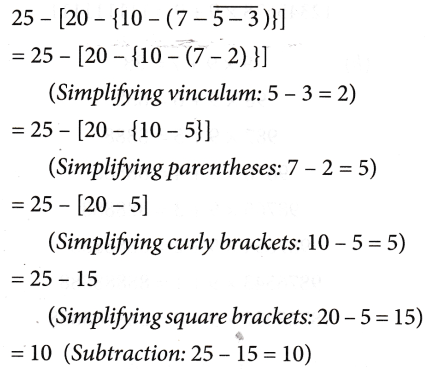RULES FOR SIMPLIFICATION

1. Order of operation: The use of brackets take us to a new order of operation. The operation inside the brackets comes before the ODMAS. There are different types of brackets already mentioned here.

2. If there is no sign between a number and the bracket, then it is implied that the operation to be performed is multiplication.
Examples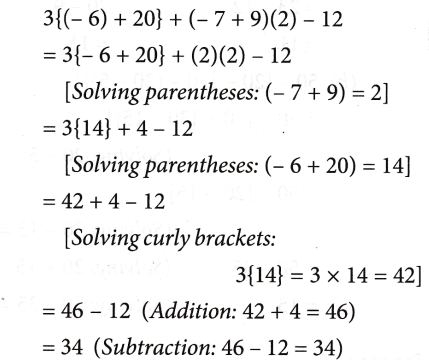3. When there is ‘+’ sign before a bracket, you can simply remove the bracket.

Examples: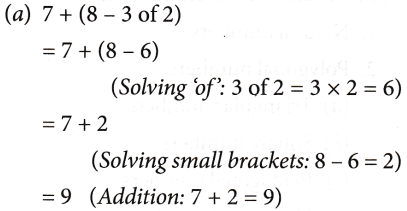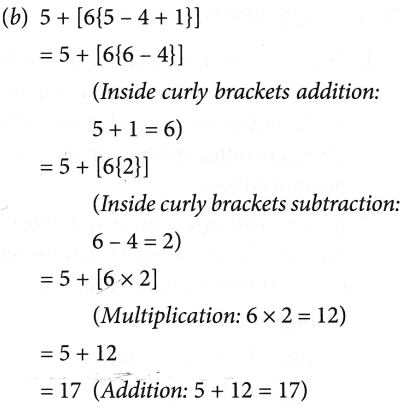4. When there is a ‘–’ sign before a bracket, then all signs within the bracket change while removing the bracket.

Examples: